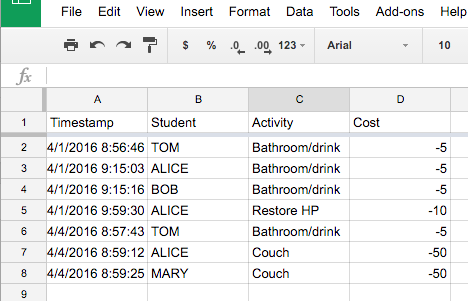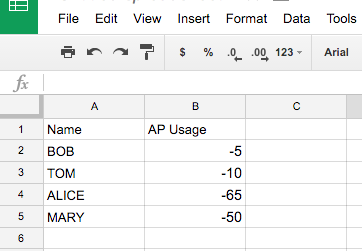I’ve been playing around with gamifying my classroom this semester. After looking at Classcraft I decided I wanted something a little simpler, so I put together a Google Sheet to keep track of XP, AP, and HP. The problem came when I needed to add or subtract a stat from a student.

# Entering the data

A Google Form was going to be the easiest, so I created a form to enter XP, AP, and HP. The first item asked for the name of the student, then a reason, and finally, the number of points. This went into a sheet in Google Sheets.But how do I add up Tom’s total number of points he spent?

For this example, the above sheet is named AP, and I have another sheet with the totals for AP. That sheet looks like this:The magic happens with the formula that is in B2:B5. The formula is:

`=if(iserror(VLOOKUP(A2,AP!\$B\$2:\$D\$523,3,FALSE)),0,sumif(AP!\$B\$2:\$B\$523,A2,AP!\$D\$2:\$D\$523))`

That looks like a mess, but lets break it down.

`=if(iserror(VLOOKUP(A2,AP!\$B\$2:\$D\$523,3,FALSE)),0`

An if statement has three parameters, the first two are above. The first parameter is the ISERROR function. This function uses the VLOOKUP function to let us know if a student’s name is actually returned in the form. Without checking for this, every student that has not used any AP will get a nice #N/A instead of a 0. If the first parameter returns true, which it would if the name isn’t sheet, then return a 0.

`sumif(AP!\$B\$2:\$B\$523,A2,AP!\$D\$2:\$D\$523))`

If the first parameter returns false, then the sumif function runs. It adds up the range AP!\$D\$2:\$D\$523 if the range AP!\$B\$2:\$B\$523 is equal to A2. And now anything entered to the AP sheet will automatically be reflected for the student totals.Be sure to subscribe to my YouTube channel and join your fellow educators on the Eduk8me email list!